1800-1023-196

+91-120-4616500

# Buffer solutions

## Buffer solutions

A solution whose pH is not altered to any great extent by the addition of small quantities of either sirong acid (H+ ions) or a sirong base (OH ions) is called the buffer solution. It can also be defined as a solution of reserve acidity or alkalinity which resists change of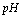upon the addition of small amount of acid or alkali.

Types of buffer solutions:

There are two types of buffer solutions,

(i) Solutions of single substances: The solution of the salt of a weak acid and a weak base.

Example: ammonium acetate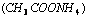,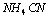act as a buffer.

(ii) Solutions of Mixtures: These are further of two types,

(a) Acidic buffer : It is the solution of a mixture of a weak acid and a salt of this weak acid with a strong base.

Example:(b) Basic buffer: It is the solution of a mixture of a weak base and a salt of this weak base with a strong acid.

Example: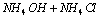(2) Buffer action: Buffer action is the mechanism by which added H+ ions or OH ions are almost neutralised; so that pH practically remains constant. Reserved base of buffer neutralises the added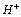ions while the reserved acid of buffer neutralises the added OH ions.

(3) Examples of buffer solutions

(i) Phthalic acid + potassium hydrogen phthalate

(ii) Citric acid + sodium citrate.

(iii) Boric acid + borax (sodium tetraborate).

(iv) Carbonic acid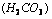+ sodium hydrogen carbonate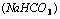. This system is found in blood and helps in maintainingof the blood close to 7.4 (value of human blood lies between 7.36 – 7.42; a change in pH by 0.2 units may cause death).

(v)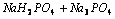(vi)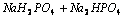(vii) Glycerine +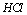(viii) Thevalue of gastric juice is maintained between 1.6 and 1.7 due to buffer system.

(4) enderson - Hasselbalch equation:of an acidic or a basic buffer can be calculated by Henderson- Hasselbalch equation.

For acidic buffers,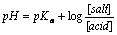When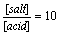, then,and

when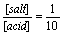, then,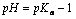So weak acid may be used for preparing buffer solutions havingvalues lying within the ranges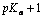and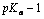. The acetic acid has a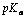of about 4.8; it may, therefore, be used for making buffer solutions withvalues lying roughly within the range 3.8 to 5.8.

For basic Buffers,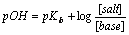Knowing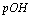,can be calculated by the application of formula,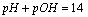of a buffer solution does not change with dilution but it varies with temperature because value of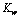changes with temperature.

(5) Buffer capacity: The property of a buffer solution to resist alteration in its pH value is known as buffer capacity. It has been found that if the ratio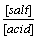or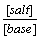is unity, the pH of a particular buffer does not change at all. Buffer capacity is defined quantitatively as number of moles of acid or base added in one litre of solution as to change the pH by unity, i.e.,

Buffer capacity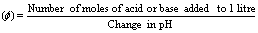Thus greater the buffer capacity, the greater is its capacity to resist change invalue. Buffer capacity is greatest when the concentration of salt and weak acid/base are equal, or when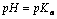or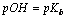.

## NEET & AIIMS Exam Sample Papers

 AIIMS SAMPLE PAPERS View More NEET SAMPLE PAPERS View More